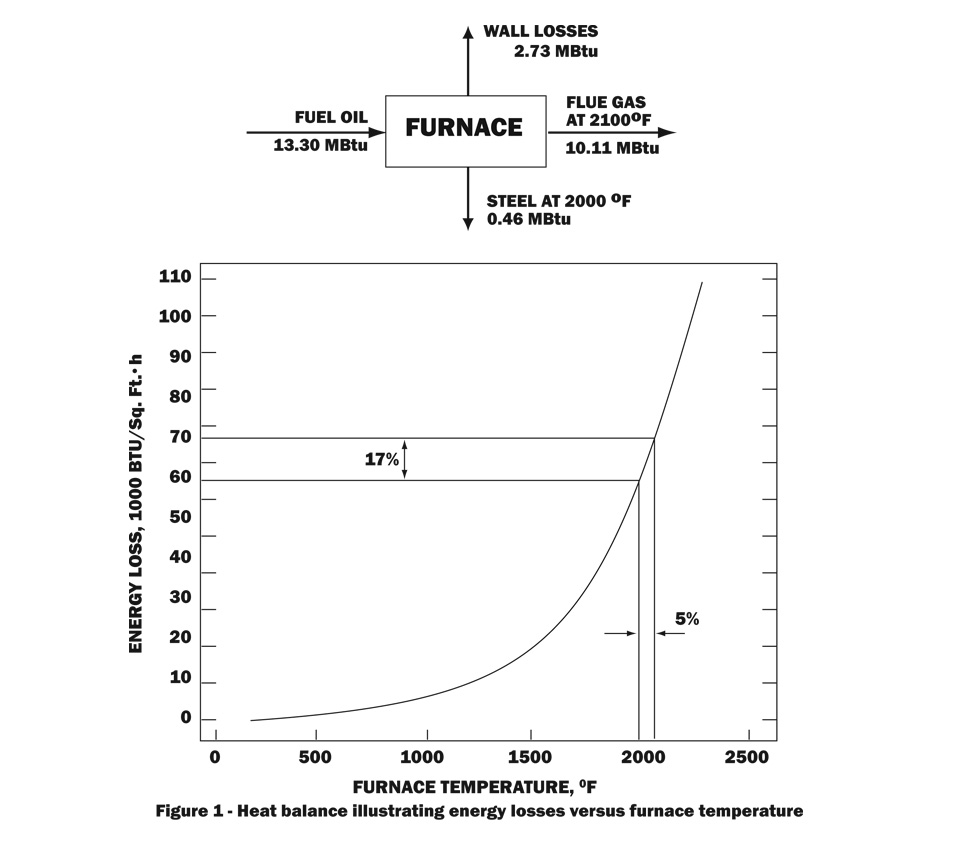# Thermal Energy Costs

Managing Energy is becoming more and more an issue due to price and availability. To calculate the required energy needed for a process, consider the energy needed to achieve process temperature, the energy lost during the time to achieve process temperature and the energy needed to maintain the process temperature. The main thing to understand is that reducing operating cost involves all 4 elements (Heatertemperature sensortemperature controller and electrical power switching) of the process.

As we all know, there is a difference between price and cost!

Here are a couple interesting articles:

The main factors that influence Thermal Energy costs are:

1. efficiency of heat transfer,
2. insulation
3. heat control

Retail prices for Energy

Different types of energy are measured by different physical units: barrels or gallons for petroleum; cubic feet for natural gas; tons for coal; kilowatt-hours for electricity. To compare different fuels, we need first convert the measurements to the same units. In the United States, the British Thermal Unit (Btu), a measure of heat energy, is the most commonly used unit for comparing fuels. Because energy used in different countries comes from different places, the Btu content of fuels varies slightly from country to country.

Based on the US Department of Energy's national average of 2006 residential retail prices, the costs are:

 Energy Source Average Retail Price Cost per million Btu Electricity \$0.1040 per kilowatt hour \$30.48 Propane \$1.88 per gallon \$20.55 No.2 Heating  Oil \$2.37 per gallon \$17.05 Natural Gas \$13.75 per thousand cubic feet \$13.35

(1 US gallon of gasoline contains roughly130'000 Btu and the price is approaching (2008) four dollars per gallon.or \$ 30.65 per million Btu)
Based on this chart, electricity appears to be the most expensive source of energy. But this chart doesn't show how efficient the energy is used.The main energy sources in factories are Natural gas (measured in BTU) and electricity (measured in Watts). To convert BTU to Watts use the conversion factor of 3.412 (1 W equals 3.412 BTU, 1kW equals 3412 BTU; 100'000 BTU equal roughly 30 kW).

 Home Basic Industrial Heating Know How Technical Calculations for Industrial Heating Thermal Energy Costs Standard and Norms Technical Papers Credit Application RFQ and Contact Us

# Thermal Energy Costs

Managing Energy is becoming more and more an issue due to price and availability. To calculate the required energy needed for a process, consider the energy needed to achieve process temperature, the energy lost during the time to achieve process temperature and the energy needed to maintain the process temperature. The main thing to understand is that reducing operating cost involves all 4 elements (Heater, temperature sensor, temperature controller and electrical power switching) of the process.

As we all know, there is a difference between price and cost!

Here are a couple interesting articles:

The main factors that influence Thermal Energy costs are:

1. efficiency of heat transfer,
2. insulation
3. heat control

Retail prices for Energy

Different types of energy are measured by different physical units: barrels or gallons for petroleum; cubic feet for natural gas; tons for coal; kilowatt-hours for electricity. To compare different fuels, we need first convert the measurements to the same units. In the United States, the British Thermal Unit (Btu), a measure of heat energy, is the most commonly used unit for comparing fuels. Because energy used in different countries comes from different places, the Btu content of fuels varies slightly from country to country.

Based on the US Department of Energy's national average of 2006 residential retail prices, the costs are:

 Energy Source Average Retail Price Cost per million Btu Electricity \$0.1040 per kilowatt hour \$30.48 Propane \$1.88 per gallon \$20.55 No.2 Heating  Oil \$2.37 per gallon \$17.05 Natural Gas \$13.75 per thousand cubic feet \$13.35

(1 US gallon of gasoline contains roughly130'000 Btu and the price is approaching (2008) four dollars per gallon.or \$ 30.65 per million Btu)
Based on this chart, electricity appears to be the most expensive source of energy. But this chart doesn't show how efficient the energy is used.The main energy sources in factories are Natural gas (measured in BTU) and electricity (measured in Watts). To convert BTU to Watts use the conversion factor of 3.412 (1 W equals 3.412 BTU, 1kW equals 3412 BTU; 100'000 BTU equal roughly 30 kW).